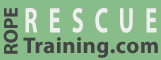The discussion about raising explained that "mechanical advantage" is a measurement of how much your rope and pulley system leverages the force than you put into it. This page explains a few terms relating to mechanical advantage. The following pages explain how to calculate mechanical advantage.

A system's mechanical advantage is expressed as a ratio using a colon. For example, a 2:1 or 3:1 system (these are pronounced as a “2 to 1" and “3 to 1"). The first number represents the force on the load and the second number is the force that the rescuers are pulling on the rope. For example, when using a 3:1 system, for every three pounds of load, the rescuers will be holding one pound. This means that the rescuers will only need to pull 100 pounds to raise a 300-pound load.